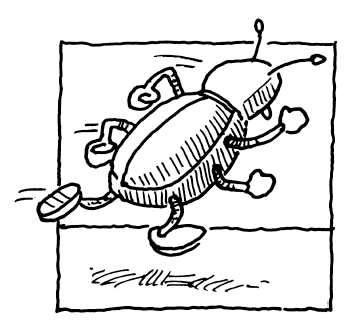### Home > PC3 > Chapter 10 > Lesson 10.2.2 > Problem10-133

10-133.A very fast bug is walking counterclockwise around the edge of a circular table with a radius of $3$ feet. At time $t = 0$ she starts at the point $(3, 0)$ and walks at a rate of $3$ ft/s.

1. Explain why the angle at which the bug has traveled to her position after $1$ second is $1$ radian.

Compare the circumference of the circle to the given rate.

2. Explain why the angle through which the bug has traveled to her position after $t$ seconds is $t$ radians.

$\frac{1 \text{ rad}}{1 \text{ s}}\cdot\frac{t}{t}=$

3. Explain why the $x$-coordinate of the bug is $3\cos(t)$ after $t$ seconds.

Since the radius of the circle is $3$, the $x$-coordinate of any point on the circle is $3\cos(θ)$.

4. What is the $y$-coordinate of the bug after t seconds?

5. Model the motion of the bug with a set of parametric equations.